Unbalanced Oxidation Reduction Reaction Questions

A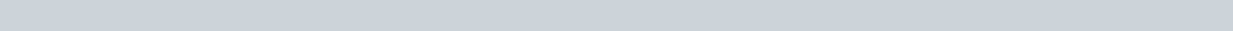AGroxnt. K for the following reaction at 25 oc is 0.28.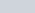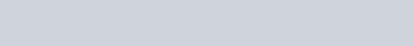(3 pt) (a) What is

1. pt) (b) Based on your answer126)

2. Consider the following unbalanced oxidation reduction reaction: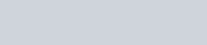Mn042-(aq) + W(s)1. pt) (a) Assign oxidation numbers to each element in the reactants and products. Write the oxidation number for that element above each elemental symbol in the above equation.(2 pt) (b) Write out the unbalanced oxidation and reduction half reactions.

Oxidation: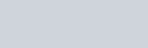Fom A

Mn042-(aq) + W(s) woe (aq) + Mn02(s) (6 pt) reaction assuming it occurs under acidic conditions.1. pt) (e) What is the complete balanced equation for the reaction in basic media?BAGroxn f AG O -RT1nK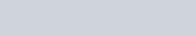R =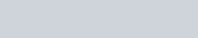kJ/(m01.K) O oc = 273.15 K

1.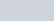pt)2. pt)(b)

2. Consider the following unbalanced oxidation reduction reaction:1. pt) (a) Assign oxidation numbers to each element in the reactants and products. Write the oxidation number for that element above each elemental symbol in the above equation.

(2 pt) (b) Write out the unbalanced oxidation and reduction half reactions.

Oxidation:B

M-(aq) S032 W(s) + SO? (aq)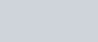reaction it under acidic conditions.(2

pt)

1. pt) (e) What is the complete balanced equation for the reaction in basic media?CAGr0xn – EG O AGr0xn —RT1nK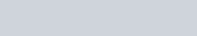R = 8.314<0-3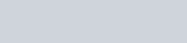O OC = 273.15 K

1.pt)
2. pt)
3. pt) (a) Assign oxidation numbers to each element in the reactants and products. Write the oxidation number for that element above each elemental symbol in the above equation.

(2 pt) (b) Write out the unbalanced oxidation and reduction half reactions.

Oxidation:€eoqt geo: (9

# -+

Fe042-(aq) Br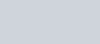reaction it Form C

-5 Fe02-(aq) + Bro-(aq)

under acidic conditions.

ba…ÅQ ee

| C)(2

1. pt) (e) What is the complete balanced equation for the reaction in basic media?D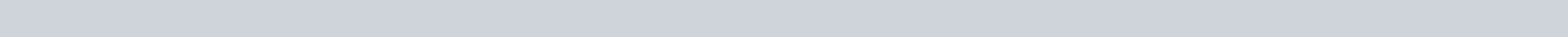f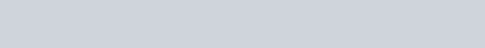O oc = 273.15 Kl.

K

for

the

following

reaction

at

25

c

is

6.5

x

lO

1. pt) (a) What is AG%nat25 c?+

12(s)

3

C12(g)

2(2

pt)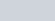2. Consider the following unbalanced oxidation reduction reaction:(3 pt) (a) Assign oxidation numbers to each element in the reactants and products. Write the oxidation number for that element above each elemental symbol in the above equation.

(2 pt) (b) Write out the unbalanced oxidation and reduction half reactions.

Oxidation:Soq -aForm D

Fe02-(aq) S042 Fe042-(aq) + S032-(aq) (6 pt) reaction it under acidic conditions.(2

(2 pt) (e) What is the complete balanced equation for the reaction in basic media?